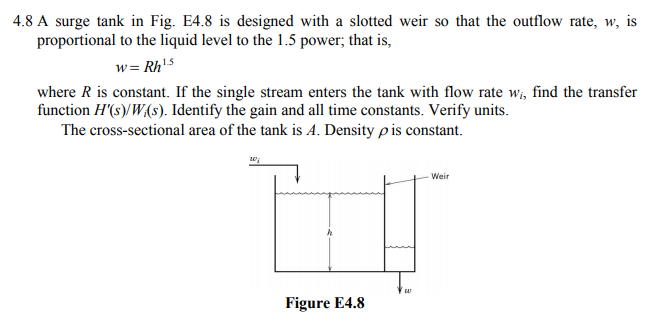4.8 A surge tank in Fig. E4.8 is designed with a slotted weir so that the outflow rate, w, isproportional to the liquid level to the 1.5 power, that is,wRh15where R is constant. If the single stream enters the tank with flow rate w, find the transferfunction H(s)/W(s). Identify the gain and all time constants. Verify unitsThe cross-sectional area of the tank is A. Density ρ is constant.-WeirFigure E4.8

Questionhelp_outlineImage Transcriptionclose4.8 A surge tank in Fig. E4.8 is designed with a slotted weir so that the outflow rate, w, is proportional to the liquid level to the 1.5 power, that is, wRh15 where R is constant. If the single stream enters the tank with flow rate w, find the transfer function H(s)/W(s). Identify the gain and all time constants. Verify units The cross-sectional area of the tank is A. Density ρ is constant. -Weir Figure E4.8 fullscreen
Step 1

Apply material balance on the given system as:

Step 2

Use Tylor series to expand the equation (1) as shown below.

Step 3

Now, take Laplace transform of equation (2) and r...

Want to see the full answer?

See Solution

Want to see this answer and more?

Our solutions are written by experts, many with advanced degrees, and available 24/7

See Solution
Tagged in

Chemical Engineering